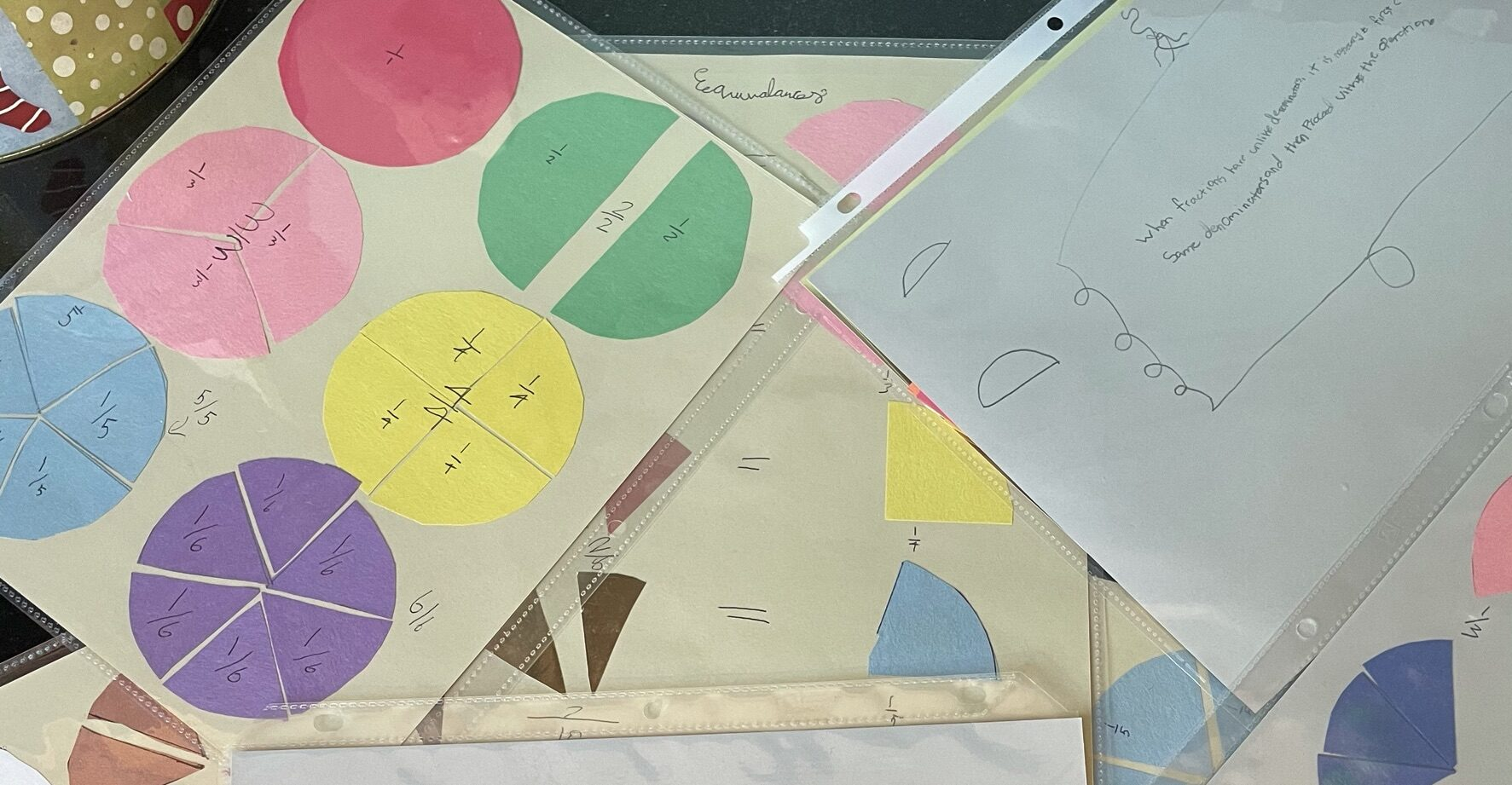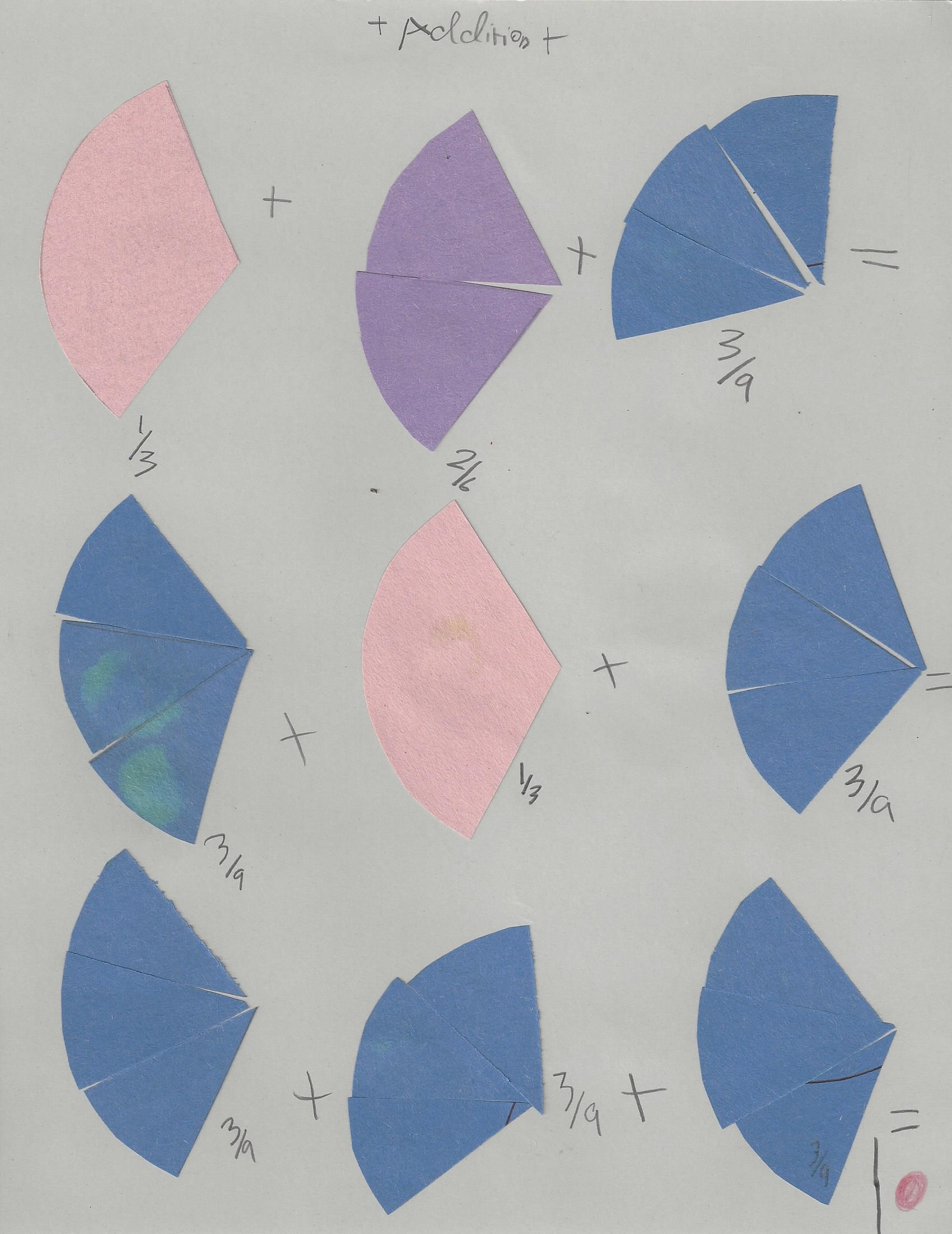# Montessori Fraction Charts

Today my oldest finished a huge project! For the past year or so, she’s been making the Montessori Fraction Charts.

The Fraction Charts are a bit like a fraction dictionary. The charts show fraction circles 1-10, fraction equivalence, fraction addition and subtraction with like and unlike denominators, multiplication of a fraction by a whole number and fraction, and division of a fraction by whole number and fraction. Each chart can be introduced after the child has abstracted and mastered the relevant concept. They are not a teaching tool, but a reference tool.

In a classroom, fraction charts will be printed or perhaps made by the guide. At home, it seemed like a good project for Fungirl to take on. Looking back at the pictures, I now realize that she began over a year ago. In the early part of the 2020-2021 school year she made the first charts demonstrating the fraction circles, equivalence, and the addition and subtraction charts.

The multiplication charts followed in the Spring of 2021, and then the division charts in the Fall of 2021. A long project, but work the revisiting and time spent. The charts have been used as a reference tool, and I think I’ll have Daisy make them too – and probably stretch the process over a longer period. She could probably make the first 4 charts, plus addition and subtraction with like denominators.

My main reference source was the What DID we do all day blog, which has unfortunately been taken down – though you can still access her amazing posts through the wayback machine. My second source were images from the guavarama website. She has a set of chart images for purchase.

When making the earlier charts, Fungirl traced around our fraction pieces and cut out the shapes. This time, to entice her to finish up, I set her up on my computer with my Fraction Circle SVG files loaded into Cricut Design Space and showed her how to select just the pieces she needed. I walked her all the way through selecting her material, loading the mat, and cutting pieces twice and then she cut just about everything else independently. It was a great additional use of my SVG files!

If you’d like to see more about our homemade fraction circles, I’ve blogged about them here. You can purchase the Fraction Circle SVG files in my store, on Etsy, or on Teachers Pay Teachers.

If you’d like to try your hand – or your child’s hand – at making the fraction charts, here’s what we settled on, for your reference:Chart 10: 1/3 + 2/6 + 3/9 = 3/9 + 1/3 + 3/9 = 3/9 + 3/9 + 3/9 = 1Chart 12: Rule for Addition & Subtraction with Unlike Denominators When fractions have unlike denominators it is necessary to first change them to the same denominators and then proceed with the operation.

The numerator is multiplied by 2:
1/4 x 2 = (1×2)/4 = 1/2
OR
The denominator is divided by 2:
1/4 x 2 = 1/(4÷2) = 1/2

Rule: When multiplying a fraction by a while number one can multiply the numerator by the whole number
OR
If divisible, divide the denominator by the whole number.

To say 1/3 x 2 means to take:

The say 1/3 x 1/2 means to take: …just half a time.

Then take two parts of each third.

Rule: To multiply a fraction by a fraction, one must multiply numerator by numerator and denominator by denominator.

2/3 x 2/3 = 4/9

Rule: To divide a fraction by a whole number, instead of dividing the numerator, one can multiply the denominator by that number.

In dividing a fraction by a fraction, one must remember that the result of a division is the amount received by each unit of the division.

Each third will receive one half of 1/4.

As the unit is formed by 3 thirds, the result will be 3/8.

Rule: To divide a fraction by a fraction, one multiplies the first fraction by the inverse of the second fraction.